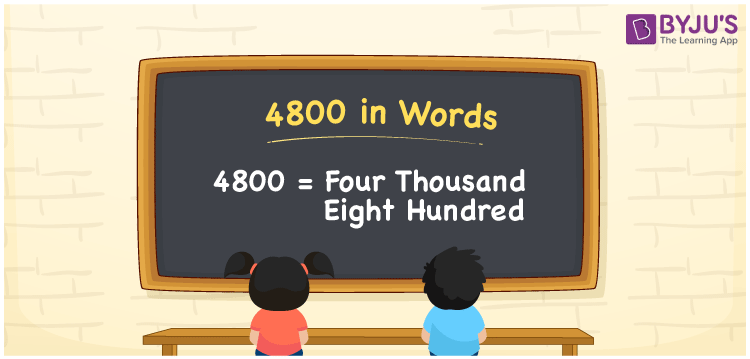# 4800 in Words

We can write 4800 in words as Four thousand eight hundred. We know that 4800 is a cardinal number that denotes a specific quantity. Suppose you spent Rs. 4800 to repair your bike, then you can say, “I spent Four thousand eight hundred rupees to repair my bike”. In this article, you will learn the simple method of writing 4800 in words.

 4800 in words Four thousand eight hundred Four thousand eight hundred in Numbers 4800

## 4800 in English Words

We generally write numbers in words with the help of the English alphabet. So, we can read 4800 in English as “Four thousand eight hundred”.## How to Write 4800 in Words?

The below table depicts the place value chart with four columns since the number 4800 contains four digits.

 Thousands Hundreds Tens Ones 4 8 0 0

Here, ones = 0, tens = 0, hundreds = 8, and thousands = 4.

Thus, we can write the expanded form as:

4 × Thousand + 8 × Hundred + 0 × Ten + 0 × One

= 4 × 1000 + 8 × 100 + 0 × 10 + 0 × 1

= 4000 + 800

= Four thousand + Eight hundred

= Four thousand eight hundred

Hence, 4800 in words = Four thousand eight hundred.

4800 is a natural number that precedes 4801 and succeeds 4799.

4800 in words – Four thousand eight hundred

Is 4800 an odd number? – No

Is 4800 an even number? – Yes

Is 4800 a prime number? – No

Is 4800 a composite number? – Yes

Is 4800 a perfect square number? – No

Is 4800 a perfect cube number? – No

## Frequently Asked Questions on 4800 in Words

Q1

### How do you write 4800 in words?

We generally write 4800 in words as Four thousand eight hundred.
Q2

### How to write 4800 in words on a cheque?

On a cheque, 4800 should be written in words as Four thousand eight hundred rupees only.
Q3

### What number is four thousand eight hundred in words?

The number 4800 is called four thousand eight hundred in words.